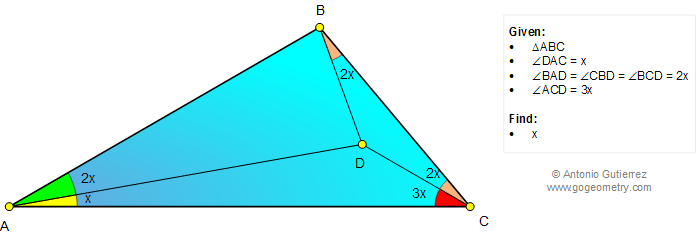# Math: Geometry Problem 1025: Triangle, Double, Triple, Angle, Interior Point. Level: School, College.

< PREVIOUS PROBLEM  |  NEXT PROBLEM >

 The figure below shows a triangle ABC with angle DAC = x, angle BAD = angle CBD = angle BCD = 2x, and angle ACD = 3x. Find x.See also: GeoGebra of problem 1025

Home | SearchGeometry | Problems | All Problems | Open Problems | Visual Index | 10 Problems | Problems Art Gallery Art | 1021-1030 | Triangle | Double Angle | Email | by Antonio Gutierrez

Add a solution to the problem 1025
Last updated: Oct 22, 2017